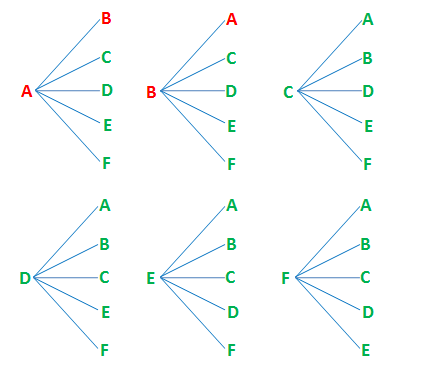Example: Handshake Problem

Question

Assume there are 10 people in a room, including you. Each person in the room must shake hands one time, and only time, with all the other people in the room. How many handshakes will occur? If there are 20 people in the room, how many handshakes will occur? If there are N (where N > 0) people in the room, how many handshakes will occur?Understanding the problem

1.Shake hands with 10/20/n people

2.Cannot shake hands with same person twice

3.Cannot shake hands with myself

Plan the solution

Numbers of people Time for handshake
1 0
2 1=1
3 2+1=3
4 3+2+1=6
5 4+3+2+1=10
6 5+4+3+2+1=15
7 6+5+4+3+2+1=21
8 7+6+5+4+3+2+1=28

Carry out the plan

(n-1)+(n-2)+(n-3)+...+ 3 + 2 + 1

+

1 + 2 + 3 +...+(n-3)+(n-2)+(n-1)

______________________________

2

= n*(n-1)/2

Review and Discussion

Result: n*(n-1)/2